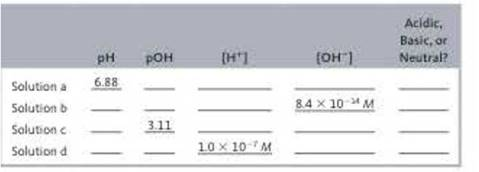Chapter 14, Problem 51E

Chapter
Section
Textbook Problem

# Fill in the missing information in the following table.Interpretation Introduction

Interpretation:

The missing information in the given table is to be stated.

Concept introduction:

The pH is the measure of its [H+] of any solution.  The pOH is the measure of the [OH] of any solution. [H+] represents the total hydrogen ion concentration. [OH] represents the total hydroxide ion concentration.  The nature of any solution is determined by its pH and pOH value.

Explanation

To determine: The missing values in the table for solution a.

The pOH is 7.12_ .

Given

The pH of the solution is 6.88 .

The sum,

pH+pOH=14

Where,

• pOH is the measure of  hyroxide ion concentration.
• pH is the measure of hydrogen ion concentration.

Substitute the value of pH in the above equation.

6.88+pOH=14pOH=146.88pOH=7.12_

The [H+] is 1.31×10-7M_ .

Given

The pH of the solution is 6.88 .

The pH value is calculated by the formula,

pH=log[H+]

Where,

• [H+] is the total concentration of hydrogen ions.

Rearrange the above equation to calculate the value of [H+] .

[H+]=antilog(pH)

Substitute the value of pH in the above equation.

[H+]=antilog(pH)[H+]=Antilog(6.88)[H+]=1.31×10-7M_

The [OH] is 7.6×10-8M_ .

Given

The [H+] is 1.31×107M .

The ionic product of water is,

[H+][OH]=1.0×1014

Where,

• [H+] is the concentration of hydrogen ion.
• [OH] is the concentration of hydroxide ion.

Substitute the value of [H+] in the above equation.

1.31×107×[OH]=1.0×1014[OH]=1.0×10141.31×107[OH]=7.6×10-8M_

The solution is acidic.

In the given solution, the [H+]>[OH] . Therefore, the given solution is acidic.

To determine: The missing values in the table for solution b

The [H+] is 0.119M_ .

Given

The [OH] is 8.4×1014 .

The ionic product of water is,

[H+][OH]=1.0×1014

Where,

• [H+] is the hydrogen ion concentration.
• [OH] is the hydroxide ion concentration.

Substitute the value of [OH] in the above equation.

[H+]×8.4×1014=1.0×1014[H+]=1.0×10148.4×1014[H+]=0.119M_

The pH is 0.92_

Given

The [H+] is 0.119M

The pH value is calculated by the formula,

pH=log[H+]

Where,

• [H+] is the total concentration of hydrogen ions.

Substitute the value of [H+] in the above equation.

pH=log[0.119]pH=0.92_

The pOH is 13.08_ .

Given

The pH is 0.92 .

The sum pH+pOH=14

Substitute the value of pH in the above equation.

0.92+pOH=14pOH=140

### Still sussing out bartleby?

Check out a sample textbook solution.

See a sample solution

#### The Solution to Your Study Problems

Bartleby provides explanations to thousands of textbook problems written by our experts, many with advanced degrees!

Get Started